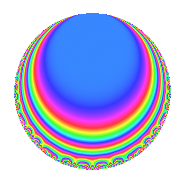# Properties

 Label 136.1.p.aLevel 136 Weight 1 Character orbit 136.p Analytic conductor 0.068 Analytic rank 0 Dimension 4 Projective image $$D_{8}$$ CM disc. -8 Inner twists 4

# Related objects

## Newspace parameters

 Level: $$N$$ = $$136 = 2^{3} \cdot 17$$ Weight: $$k$$ = $$1$$ Character orbit: $$[\chi]$$ = 136.p (of order $$8$$ and degree $$4$$)

## Newform invariants

 Self dual: No Analytic conductor: $$0.0678728417181$$ Analytic rank: $$0$$ Dimension: $$4$$ Coefficient field: $$\Q(\zeta_{8})$$ Coefficient ring: $$\Z[a_1, a_2]$$ Coefficient ring index: $$1$$ Projective image $$D_{8}$$ Projective field Galois closure of 8.0.1680747204608.3

## $q$-expansion

The $$q$$-expansion and trace form are shown below.

 $$f(q)$$ $$=$$ $$q$$ $$+ \zeta_{8}^{3} q^{2}$$ $$+ ( \zeta_{8}^{2} - \zeta_{8}^{3} ) q^{3}$$ $$-\zeta_{8}^{2} q^{4}$$ $$+ ( -\zeta_{8} + \zeta_{8}^{2} ) q^{6}$$ $$+ \zeta_{8} q^{8}$$ $$+ ( -1 + \zeta_{8} - \zeta_{8}^{2} ) q^{9}$$ $$+O(q^{10})$$ $$q$$ $$+ \zeta_{8}^{3} q^{2}$$ $$+ ( \zeta_{8}^{2} - \zeta_{8}^{3} ) q^{3}$$ $$-\zeta_{8}^{2} q^{4}$$ $$+ ( -\zeta_{8} + \zeta_{8}^{2} ) q^{6}$$ $$+ \zeta_{8} q^{8}$$ $$+ ( -1 + \zeta_{8} - \zeta_{8}^{2} ) q^{9}$$ $$+ ( -1 + \zeta_{8}^{3} ) q^{11}$$ $$+ ( 1 - \zeta_{8} ) q^{12}$$ $$- q^{16}$$ $$-\zeta_{8}^{3} q^{17}$$ $$+ ( -1 + \zeta_{8} - \zeta_{8}^{3} ) q^{18}$$ $$+ ( -\zeta_{8}^{2} - \zeta_{8}^{3} ) q^{22}$$ $$+ ( 1 + \zeta_{8}^{3} ) q^{24}$$ $$-\zeta_{8} q^{25}$$ $$+ ( 1 - \zeta_{8} - \zeta_{8}^{2} + \zeta_{8}^{3} ) q^{27}$$ $$-\zeta_{8}^{3} q^{32}$$ $$+ ( -\zeta_{8} + \zeta_{8}^{3} ) q^{33}$$ $$+ \zeta_{8}^{2} q^{34}$$ $$+ ( -1 + \zeta_{8}^{2} - \zeta_{8}^{3} ) q^{36}$$ $$+ ( \zeta_{8} + \zeta_{8}^{2} ) q^{41}$$ $$+ ( 1 + \zeta_{8}^{2} ) q^{43}$$ $$+ ( \zeta_{8} + \zeta_{8}^{2} ) q^{44}$$ $$+ ( -\zeta_{8}^{2} + \zeta_{8}^{3} ) q^{48}$$ $$+ \zeta_{8}^{3} q^{49}$$ $$+ q^{50}$$ $$+ ( \zeta_{8} - \zeta_{8}^{2} ) q^{51}$$ $$+ ( 1 + \zeta_{8} - \zeta_{8}^{2} + \zeta_{8}^{3} ) q^{54}$$ $$+ ( -1 - \zeta_{8}^{2} ) q^{59}$$ $$+ \zeta_{8}^{2} q^{64}$$ $$+ ( 1 - \zeta_{8}^{2} ) q^{66}$$ $$+ ( \zeta_{8} - \zeta_{8}^{3} ) q^{67}$$ $$-\zeta_{8} q^{68}$$ $$+ ( -\zeta_{8} + \zeta_{8}^{2} - \zeta_{8}^{3} ) q^{72}$$ $$+ ( \zeta_{8}^{2} + \zeta_{8}^{3} ) q^{73}$$ $$+ ( -1 - \zeta_{8}^{3} ) q^{75}$$ $$+ ( -\zeta_{8} + \zeta_{8}^{2} - \zeta_{8}^{3} ) q^{81}$$ $$+ ( -1 - \zeta_{8} ) q^{82}$$ $$+ ( 1 - \zeta_{8}^{2} ) q^{83}$$ $$+ ( -\zeta_{8} + \zeta_{8}^{3} ) q^{86}$$ $$+ ( -1 - \zeta_{8} ) q^{88}$$ $$+ ( -\zeta_{8} - \zeta_{8}^{3} ) q^{89}$$ $$+ ( \zeta_{8} - \zeta_{8}^{2} ) q^{96}$$ $$+ ( -1 + \zeta_{8} ) q^{97}$$ $$-\zeta_{8}^{2} q^{98}$$ $$+ ( \zeta_{8}^{2} - \zeta_{8}^{3} ) q^{99}$$ $$+O(q^{100})$$ $$\operatorname{Tr}(f)(q)$$ $$=$$ $$4q$$ $$\mathstrut -\mathstrut 4q^{9}$$ $$\mathstrut +\mathstrut O(q^{10})$$ $$4q$$ $$\mathstrut -\mathstrut 4q^{9}$$ $$\mathstrut -\mathstrut 4q^{11}$$ $$\mathstrut +\mathstrut 4q^{12}$$ $$\mathstrut -\mathstrut 4q^{16}$$ $$\mathstrut -\mathstrut 4q^{18}$$ $$\mathstrut +\mathstrut 4q^{24}$$ $$\mathstrut +\mathstrut 4q^{27}$$ $$\mathstrut -\mathstrut 4q^{36}$$ $$\mathstrut +\mathstrut 4q^{43}$$ $$\mathstrut +\mathstrut 4q^{50}$$ $$\mathstrut +\mathstrut 4q^{54}$$ $$\mathstrut -\mathstrut 4q^{59}$$ $$\mathstrut +\mathstrut 4q^{66}$$ $$\mathstrut -\mathstrut 4q^{75}$$ $$\mathstrut -\mathstrut 4q^{82}$$ $$\mathstrut +\mathstrut 4q^{83}$$ $$\mathstrut -\mathstrut 4q^{88}$$ $$\mathstrut -\mathstrut 4q^{97}$$ $$\mathstrut +\mathstrut O(q^{100})$$

## Character Values

We give the values of $$\chi$$ on generators for $$\left(\mathbb{Z}/136\mathbb{Z}\right)^\times$$.

 $$n$$ $$69$$ $$103$$ $$105$$ $$\chi(n)$$ $$-1$$ $$-1$$ $$\zeta_{8}$$

## Embeddings

For each embedding $$\iota_m$$ of the coefficient field, the values $$\iota_m(a_n)$$ are shown below.

For more information on an embedded modular form you can click on its label.

Label $$\iota_m(\nu)$$ $$a_{2}$$ $$a_{3}$$ $$a_{4}$$ $$a_{5}$$ $$a_{6}$$ $$a_{7}$$ $$a_{8}$$ $$a_{9}$$ $$a_{10}$$
19.1
 0.707107 − 0.707107i 0.707107 + 0.707107i −0.707107 − 0.707107i −0.707107 + 0.707107i
−0.707107 0.707107i 0.707107 0.292893i 1.00000i 0 −0.707107 0.292893i 0 0.707107 0.707107i −0.292893 + 0.292893i 0
43.1 −0.707107 + 0.707107i 0.707107 + 0.292893i 1.00000i 0 −0.707107 + 0.292893i 0 0.707107 + 0.707107i −0.292893 0.292893i 0
59.1 0.707107 0.707107i −0.707107 + 1.70711i 1.00000i 0 0.707107 + 1.70711i 0 −0.707107 0.707107i −1.70711 1.70711i 0
83.1 0.707107 + 0.707107i −0.707107 1.70711i 1.00000i 0 0.707107 1.70711i 0 −0.707107 + 0.707107i −1.70711 + 1.70711i 0
 $$n$$: e.g. 2-40 or 990-1000 Significant digits: Format: Complex embeddings Normalized embeddings Satake parameters Satake angles

## Inner twists

Char. orbit Parity Mult. Self Twist Proved
1.a Even 1 trivial yes
8.d Odd 1 CM by $$\Q(\sqrt{-2})$$ yes
17.d Even 1 yes
136.p Odd 1 yes

## Hecke kernels

There are no other newforms in $$S_{1}^{\mathrm{new}}(136, [\chi])$$.